# 学习中心

Course:

Modeling with Partial Differential Equations in COMSOL Multiphysics

## Modeling with PDEs: Convection–Diffusion Equations

In Part 3 of this course on modeling with PDEs, we will expand on the techniques demonstrated in Part 2 for modeling with diffusion equations and discuss using the Coefficient Form PDE interface for modeling with convection–diffusion equations.

### The Continuity Equation

By analyzing the continuity equation for mass conservation, we will better understand the origin of some of the terms in the Coefficient Form PDE. Let us first review the continuity equation for mass transport in a fluid: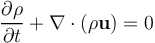where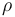is some mass density and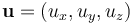is the velocity of the fluid, which may come from the solution of a fluid equation(s) such as the Navier–Stokes equations. This continuity equation describes conservation of mass where the transport is by convection.

The continuity equation can also be formulated for mass transport of a chemical species. In this case, instead of mass densitywe have the molar density, or concentration,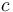. The equation then takes the following form, in the absence of reactions: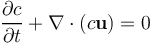If reactions are included, the equation would instead be: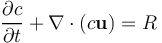where the term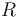is called the reaction term. Here, to simplify matters, we will assume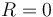.

The quantity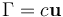is the mass (or molar) flux. For stationary transport, we have that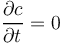and then the continuity equation reads: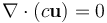Another way to approach modeling of mass conservation is to use the integral form of the continuity equation over a closed surface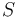: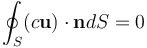where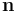is the unit surface normal, or, more generally in terms of flux: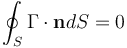This relationship states that mass flux is conserved, or balanced, over a closed surface,. In other words: "mass that goes into a control volume from one side must come out on the other side".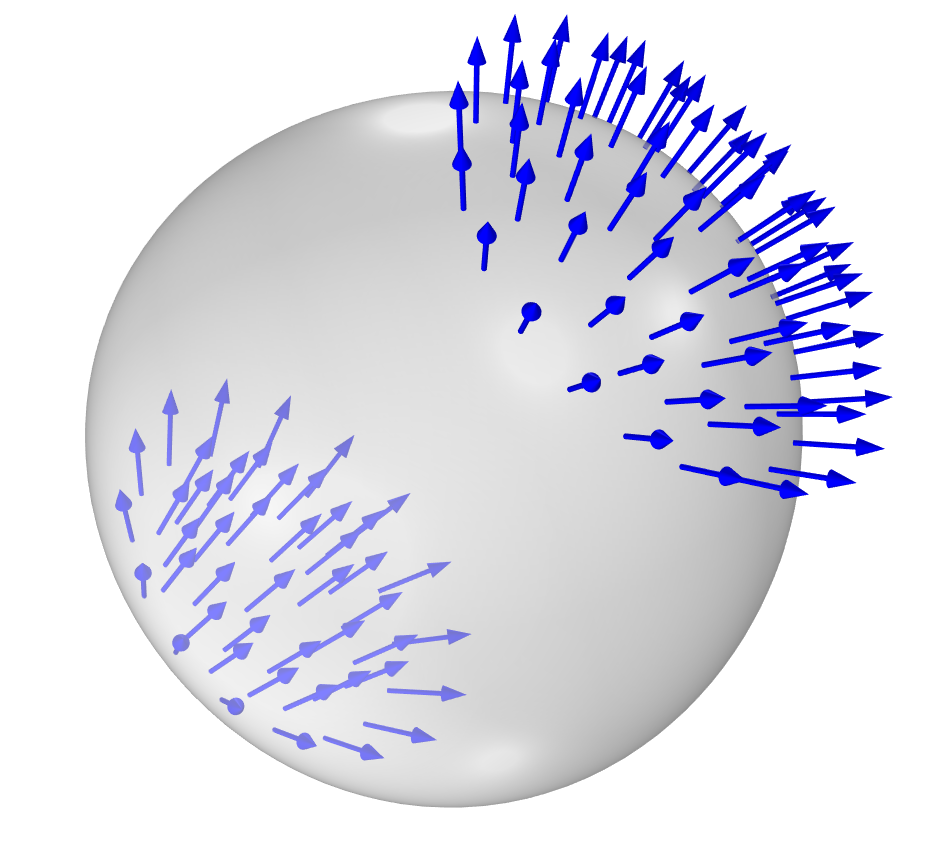Roughly speaking, the stationary continuity equation states that any flux that enters a control volume from one side needs to exit on the other side.

When we add the time-derivative term we can also account for the rate of change over time.

Note that in addition to mass and concentration, conservation equations can be formulated for many other different physical quantities, such as the conservation of charge or heat. For more information on conservation equations, click here.

### Convective and Diffusive Flux

There is frequently a diffusive contribution to the mass flux: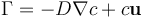where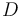is a diffusion coefficient.

In this case, the continuity equation reads: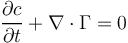or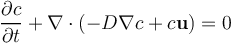A microchannel mixer model in the rainbow color table with a slice through the middle.
Diffusive flux (red arrows) and convective flux (blue arrows) in a microchannel mixer. The concentration of a species is visualized by a colored slice going through the middle of the channel. The diffusive flux is directed from regions of high concentration toward regions of low concentration. Here, the convective flux comes from the solutions of the Navier–Stokes equations.

There may be two different kinds of convective terms depending on if the fluid is compressible or not. To see this, let us take the divergence of the convective term: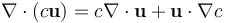For an incompressible fluid we have that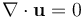, so: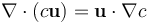In the Coefficient Form PDE, the term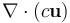is called a conservative convective term while the term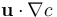is called a nonconservative convective term, or just convective term.

### The Coefficient Form PDE for Convection–Diffusion

Let us now compare the following terms to those of the Coefficient Form PDE, formulated with the concentration variableas the dependent variable: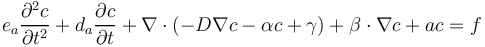We see that for mass transport described by the continuity equation: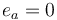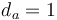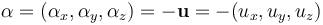or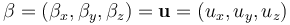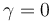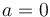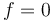If there is a chemical reaction term, then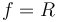Note that in the case of a possibly compressible fluid, we use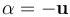and if we know the fluid is incompressible we can instead use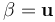. Note that you need to pick one of these two coefficients to represent convection.

For the Coefficient Form PDE, there are two important boundary conditions. The first is the generalized Neumann boundary condition: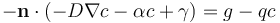,

which is used to model a boundary flux. The second is the Dirichlet boundary condition: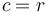which is used to assign fixed values of the dependent variable — in this case the concentration — on the boundary. We can see that for the Neumann condition, the mass flux is "inherited" from the PDE to this boundary condition and that it specifies the mass flux on the boundary.

The conservative source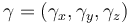provides a way of representing a source that is a vector-valued quantity, which is more suitable than the scalar source term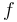, for certain applications. For example, when using the Electric Currents interface, the conservative source corresponds to an externally generated electric current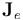in the conservation of currents PDE: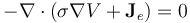Another case where the conservative source term is used is when modeling porous media flow. In this case, gravity enters the equation in a natural way as an external source term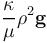in the Darcy's law PDE, as follows: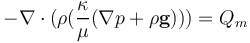Sometimes the conservative source stems from the equation of another physics represented by another dependent variable. For example, in the case of structural mechanics, this term may correspond to volumetric strain due to temperature or humidity differences.

### Numerical Stabilization

The convection–diffusion equation is interesting from a numerical analysis perspective since it is challenging to solve. There will be numerical instabilities if there is too much convection compared to diffusion. To remedy this, we can add artificial diffusion in various ways. The simple way is to just add more diffusion by increasing the value of the diffusion coefficient; however, this adds diffusion in all directions of the computational domain, also known as isotropic artificial diffusion. The drawback with this method is that, although it stabilizes the equation, it changes the solution a bit too much, so we are essentially no longer solving the original equation. Numerical analysis theory tells us that it is better to add anisotropic rather than isotropic artificial diffusion. The diffusion added in the direction of the velocity field,or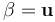, is called streamline diffusion and the diffusion added perpendicular to this velocity is called crosswind diffusion. Theory then tells us to add more streamline diffusion than crosswind diffusion. For more information, see our blog post on understanding stabilization methods.

Stabilization is done automatically and in a mathematically consistent way if, under the Mathematics interfaces, you select the Stabilized Convection-Diffusion Equation interface, as shown below in the Model Wizard. Furthermore, stabilization is also automatic if you use one of the many built-in physics interfaces for transport (such as the Transport of Diluted Species interface) or fluid flow. The stabilization methods are implemented so that the finer the mesh, the less artificial diffusion is added. In this way, as you refine the mesh, the solution converges to the solution of the original equation.The Add Physics window in COMSOL Multiphysics with the Stabilized Convection-Diffusion Equation interface selected on the left and the Add Physics window with the Transport of Diluted Species interface selected on the right.
The Stabilized Convection-Diffusion Equation interface (left) and the Transport of Diluted Species interface (right).

### Nonlinear Equations: Solving the Eikonal Equation

Let us now see how we can solve a nonlinear convection–diffusion PDE. As an example of this, let us solve the eikonal equation for computing the distance to a boundary. This can be done in a more sophisticated and robust way than what we will do here by using a different version of the eikonal equation. If we wanted to, we could implement this more robust, but also more complicated, equation using the PDE interfaces. However, it is already available as built-in functionality in the Wall Distance interface, which is used in several of the fluid flow interfaces for various distance-related calculations. For more information, see our blog post that outlines tips for using the Wall Distance interface.

Here, we will keep things simple. First, assume that within a computational domain we can represent the distance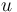to a surface as the solution to a PDE. It turns out that, at least in the vicinity of a reasonably smooth boundary, the eikonal equation can be used for computing the distanceto a boundary: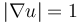If we use the Dirichlet condition: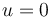on a boundary, we can interpret this as the distance being equal to 0. Then, at any point in the interior or exterior,will be the shortest distance to the boundary. However, this is true only if we are "close enough" to the boundary. What we mean by "close enough" depends on the geometry. When we go far away from the boundary we may eventually get to a point where there are multiple solutions to this equation, at which point this strategy breaks down. At such points we can say that the "phase fronts" formed by the isocontours, or isosurfaces, ofcollide. The term "phase front" comes from the fact that there is an optical interpretation to the solution of the eikonal equation.

How can we formulate the eikonal equation using one of the PDE templates in the COMSOL Multiphysics® software?

There are multiple ways. One approach is based on the fact that we can view the gradient field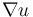as the velocity field of a fluid. If we take the square of the PDE we get: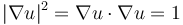We can identify this equation with the following version of the Coefficient Form PDE: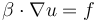where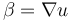and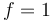We can see that in this case, the velocity field comes from the gradient of the dependent variable itself. The equation is referencing itself; this means we have a nonlinear PDE. When using the PDE templates in COMSOL Multiphysics®, you can formulate a vast number of nonlinear PDEs. Nonlinear PDEs are usually much harder to solve than linear PDEs. It is even the case that many of them do not have a solution and may not even correspond to reasonable physical phenomena. The eikonal equation is no exception, and it can be difficult to solve unless you use some special-purpose methods, as described earlier when using the built-in Wall Distance interface.

In our example, we are trying to solve the eikonal equation using the general-purpose PDE templates and we can expect at least two problems:

• As we learned earlier, a convection equation is typically unstable unless we add some diffusion
• When the phase fronts collide we do not have a unique solution

The easiest way to solve these problems is to add some artificial isotropic diffusion. This is sometimes referred to as a viscosity method. We can do this by assigning a diffusion coefficient that is not so large that it ruins the quality of the solution. However, we will have to be content with only getting an approximate solution to the eikonal equation. We will end up solving the equation: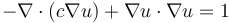We can make the amount of diffusion needed proportional to the element size. The element size is available as a built-in variable,`h`, and we can use this in expressions for the diffusion coefficient. The smaller the element size, the less diffusion we need to add, and the closer the solution will be to that of the original eikonal equation.

The theory for numerical stabilization with isotropic diffusion tells us (see Understanding Stabilization Methods) that the amount of isotropic diffusionthat we need in order to avoid oscillations is: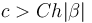where, in our case,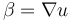and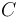is a constant that depends on the element order and how rapid the mesh element size varies. In the case of the eikonal equation we have that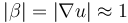so that the relationship simplifies to: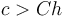However, for general nonlinear equations and geometry configurations, it can be difficult to prove that this strategy will work and to figure out how much artificial diffusion should be added. The settings used here are displayed in the screenshot below. A value of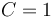is used. Since the diffusion coefficientis unitless, the unit conversion 1/m is used to convert from `h`, which has the unit of length, to a unitless quantity.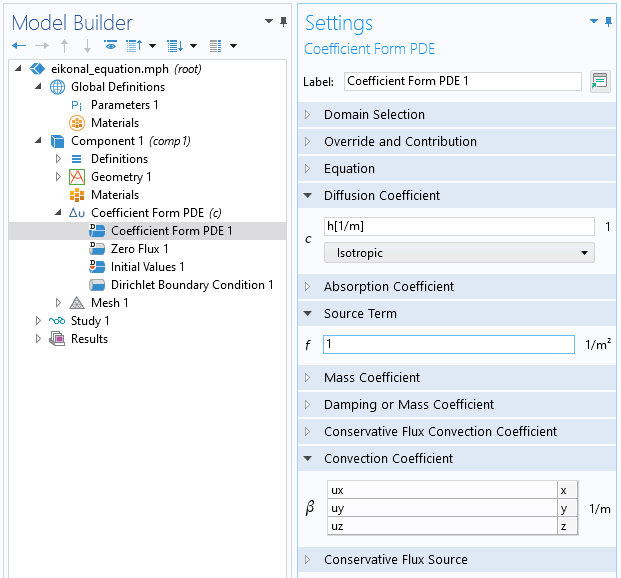The Coefficient Form PDE settings for solving the eikonal equation using a direct approach.

The Dirichlet Boundary Condition is applied on some boundaries, as seen in the figure below.The COMSOL Multiphysics UI showing Dirichlet Boundary Condition 1 selected in the Model Builder, the corresponding Settings window, and a test geometry in the Graphics window. Using the Dirichlet Boundary Condition for the eikonal equation.

The phase front corresponding to a certain distance from the boundary, or wall, can be retrieved by using an isosurface or a filter dataset, as shown below. (For more information on using these datasets, see this blog post). Thus, this method can be used for generating offset surfaces. An isosurface can be exported as an STL, PLY, or 3MF file and can potentially be used for geometry import for a different simulation.The COMSOL Multiphysics UI showing Isosurface 1 selected in the Model Builder, the corresponding Settings window, and a test geometry with a colored slice plot in the Graphics window. The solution to the eikonal equation in a test geometry that is about 50 length units long, including objects of varying curvature. In this visualization, a distance of 1.0 was used to generate the gray offset isosurface. The colored slice plot shows the solution.

### Further Learning

The model file associated with this article contains an MPH-file with the solution to the eikonal equation using the Coefficient Form PDE. In order to solve quicker and more efficiently, an iterative GMERS solver is used with a multigrid preconditioner. As an exercise, you can compare the result with that of solving with the built-in Wall Distance interface. You will discover that the results are in good agreement. Download the model file attached to this article to get started.